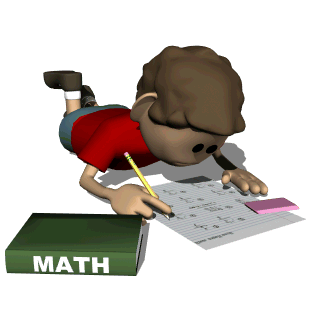•## A first grade student will be able to:

• represent numbers 1-20 in a variety of ways
• explore number sense concepts including ones and tens
• skip counting
• problem solving strategies by acting it out or drawing
• understand matematical terms related to adding and subtracting
• Learn subtraction strategies
• identify coins and uses in real life

Unit 1- Number Sense
Unit 2- Money (Mini Unit)
Unit 3- Time (Mini Unit)
Unit 5 - Subtraction
Unit 6 - Data Analysis and Measurement
Unit 7- Geometry
Unit 8- Fractions

A second grade student will be able to:
• Create number stories, model, represent, and use appropriate strategies to recall addition/subtraction facts to 24.
• Create number stories, model, represent and use appropriate strategies to compute double digit addition/subtraction problems.
• Recognize, know values and equivalences, and model combinations of coins that equal given amounts or give change for amounts up to \$1.00.
• Build models, and identify and compare attributes among various 3 dimensional shapes.
• Build models, represent and compare two and three digit numbers.
• Measure, estimate, use and compare non-standard and standard units in context.
• Represent, analyze, interpret and make predictions based on a set of contrived or student generated data